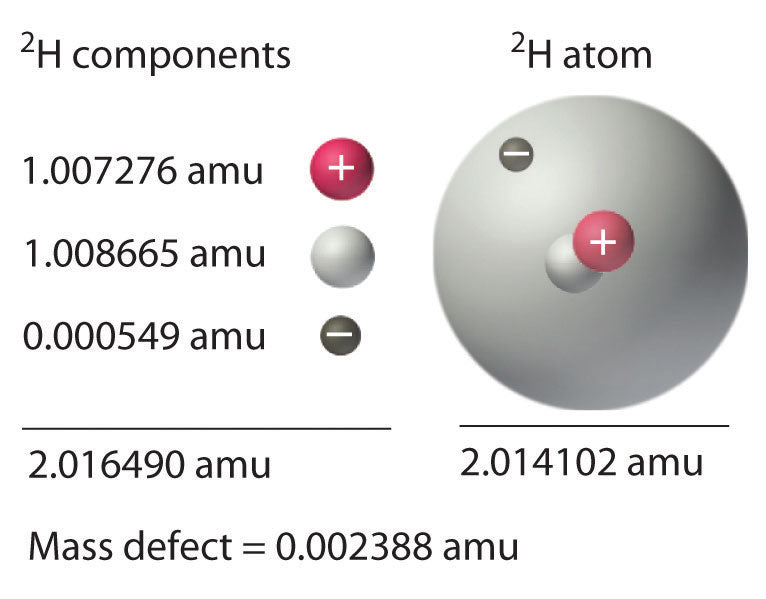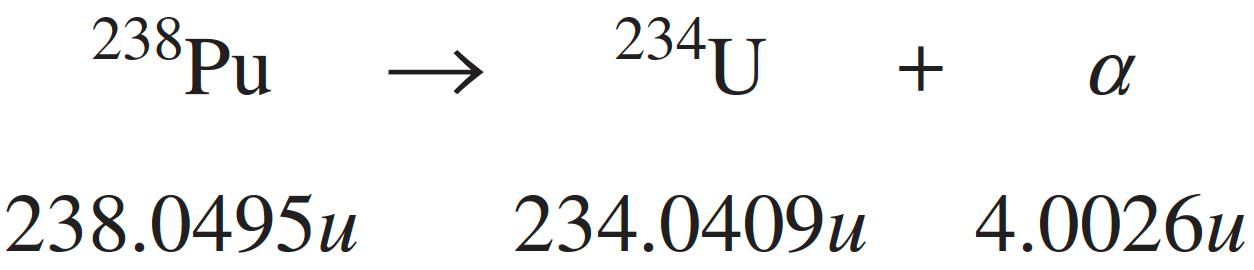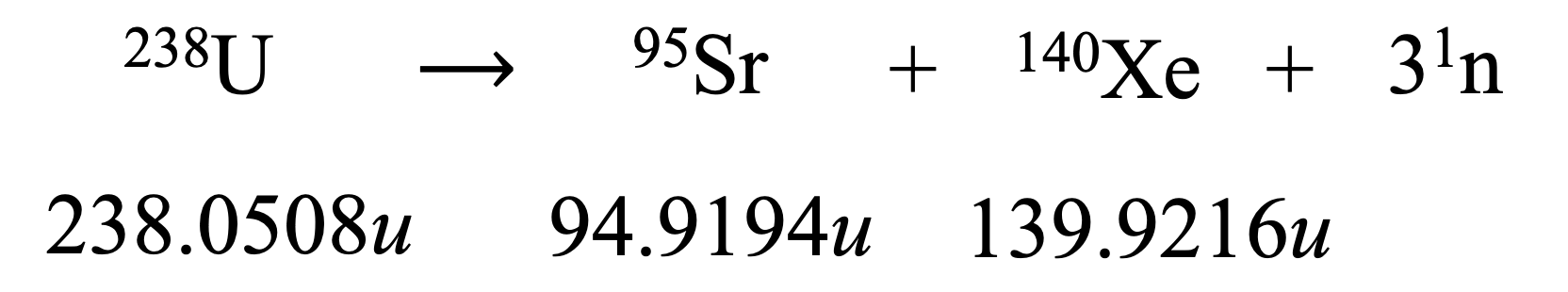# Mass Defect and Binding Energy

This topic is part of the HSC Physics course under the section Properties of the Nucleus.

### HSC Physics Syllabus

• predict quantitatively the energy released in nuclear decays or transmutations, including nuclear fission and nuclear fusion, by applying: (ACSPH031, ACSPH035, ACSPH036)

– the law of conservation of energy
– mass defect
– binding energy
– Einstein’s mass–energy equivalence relationship

### What is Mass Defect?

Mass defect is the difference between the actual mass of a nucleus and the total mass of nucleons. The total mass of nucleons is greater than the actual nuclear mass.• For a nucleus, the mass defect is the difference between the total mass of its nucleons and its actual nuclear mass.
• For an atom, the mass defect is the difference between the total mass of nucleons as well as electrons as its actual atomic mass.

### What is Binding Energy?

Mass defect is transformed into binding energy of the nucleus or atom.

Binding energy of a nucleus is the energy required to break apart the nucleons. The relationship between mass defect and binding energy is given by Einstein's energy-mass equivalence equation:

$$E_{binding}=m_{defect}c^2$$

When a nucleus/atom is formed, its binding energy is released. Conversely, when a nucleus/atom is broken apart, its binding energy is absorbed.

Binding energy per nucleon equals to the total binding energy of a nucleus divided by the number of nucleons in the nucleus.Binding energy per nucleon varies with nuclide - generally increases up to iron (Fe) and decreases after iron.

### Mass Defect and Binding Energy Calculation

Units for mass and energy:

• When mass defect is in kg, the calculated binding energy will be in J.
• When mass defect is in atomic mass unit (u), the calculated binding energy will be in MeV (by multiplying by 931.5).

For example, the binding energy of carbon-12 (nuclide mass = 12.0000 u) nucleus can be calculated:

First, calculate total mass of nucleons (6 protons and 6 neutrons)

$$m_{total} = 6 \times 1.673 \times 10^{-27} + 6 \times 1.675 \times 10^{-27}$$

$$m_{total} = 2.009 \times 10^{-26} \hspace{0.1cm} kg$$

Calculate mass defect:

$$m_{defect} = 2.009 \times 10^{-26} - 12.0000 \times 1.661 \times 10^{-27}$$

$$m_{defect} = 1.56 \times 10^{-28} \hspace{0.1cm} kg$$

Apply energy-mass equivalence equation:

$$E=mc^2$$

$$E= (1.56 \times 10^{-28})(3 \times 10^8)^2$$

$$E=1.4 \times 10^{-11} \hspace{0.1cm} J$$

Calculating binding energy per nucleon (12 nucleons in C-12):

$$E = \frac{1.4 \times 10^{-11}}{12}$$

$$E=1.2 \times 10^{-12} \hspace{0.1cm} J$$

### Binding Energy in Transmutation Reactions

Nuclear transmutation is the process through which the number of protons and/or neutrons of a nuclide changes. The total binding energy of the products of a transmutation is always different to that of the reactants.

Examples of nuclear transmutation include:

Binding energy of reactants is the amount of energy required (to be absorbed) to break apart the nuclides. Binding energy of products is the amount of energy released when the nuclides are formed.

Therefore:

• When total binding energy or products is greater than reactants, this results in an exothermic reaction (energy is released). This is because less binding energy is absorbed to break apart the reactants and more binding energy is released when products are formed.
• When total binding energy or products is less than reactants, this results in an endothermic reaction (energy is absorbed). This is because more binding energy is absorbed to break apart the reactants and less binding energy is released when products are formed.

Since binding energy per nucleon increases from hydrogen to iron, fusion reactions up to iron are all exothermic (products have greater binding energy than reactants).

Since binding energy per nucleon decreases for nuclides heavier than iron, fission of these nuclides are all exothermic (products have smaller in mass but have greater binding energy than reactants).

### Energy Produced in Radioactive Decay

The mass difference between the products and reactants of radioactive decay is also attributed to energy. The total mass of products is generally smaller than the parent nuclide. Thus, the energy produced from radioactive decay can be calculated by first determining this mass difference.

For example, alpha decay of Pu-238:Calculate mass difference:

$$\Delta m = 238.0495 - 234.0409 - 4.0026 = 0.006 u$$

$$\Delta m = 0.006 \times 1.661 \times 10^{-27} = 9.966 \times 10^{-30} \hspace{0.1cm} kg$$

Apply mass-energy equivalence equation:

$$E=mc^2$$

$$E=(9.966 \times 10^{-30})(3 \times 10^8)^2$$

$$E=9.0 \times 10^{-13} \hspace{0.1cm} J$$

### Energy Produced in Nuclear Fusion

Calculation related to nuclear fusion can be found here.

### Energy Produced in Nuclear Fission

Mass-energy equivalence and the concept of binding energy are also evident in nuclear fission reactions.

• The total mass of products will be less than that of reactants.
• The binding energy of products will be greater than that of reactants.
• This implies that energy must have been emitted in the splitting process (increase in binding energy) - this is the energy release from fission.

For example, U-238 undergoes fission to form Sr-95, Xe-140 and 3 neutrons:Calculate the mass difference:

$$m_{neutron} = \frac{1.675 \times 10^{-27}}{1.661 \times 10^{-27}} = 1.0084u$$

$$\Delta m = 238.0508 - 94.9194 -139.9216 - 3(1.0084)$$

$$\Delta m = 0.1845 u$$

Apply mass-energy equivalence equation:

$$E=mc^2$$

$$E=(0.1845 \times 931.5 MeV/c^2)(c^2)$$

$$E=172 \hspace{0.1cm} MeV$$

Previous section: Modelling Radioactive Decay (Calculation)

Next section: The Standard Model of Matter